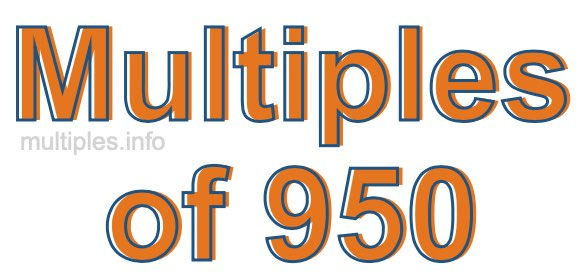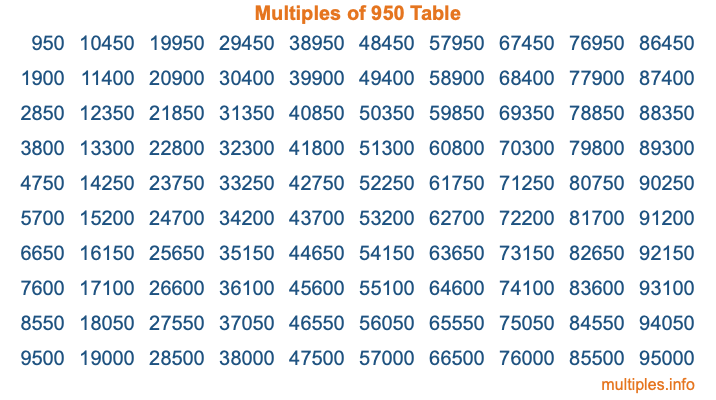Multiples of 950Welcome to the Multiples of 950 page. Here we will first teach you everything you will ever need to know about the multiples of 950, and then give you a study guide summary of everything we taught you to make sure you remember it all. Use this page to look up facts and learn information about the multiples of 950. This page will make you a multiples of nine hundred fifty expert!

Definition of Multiples of 950
Multiples of 950 are all the numbers that when divided by 950 equal an integer. Each of the multiples of 950 are called a multiple. A multiple of 950 is created by multiplying 950 by an integer.

Therefore, to create a list of multiples of 950, you start with 1 multiplied by 950, then 2 multiplied by 950, then 3 multiplied by 950, and so on for as long as you want. Thus, the list of the first five multiples of 950 is 950, 1900, 2850, 3800, and 4750. To see a larger list of multiples of 950, see the printable image of Multiples of 950 further down on this page. We also have a category where you can choose any nth multiple of 950.

Multiples of 950 Checker
The Multiples of 950 Checker below checks to see if any number of your choice is a multiple of 950. In other words, it checks to see if there is any number (integer) that when multiplied by 950 will equal your number. To do that, we divide your number by 950. If the the quotient is an integer, then your number is a multiple of 950.

Is  a multiple of 950?

Least Common Multiple of 950 and ...
A Least Common Multiple (LCM) is the lowest multiple that two or more numbers have in common. This is also called the smallest common multiple or lowest common multiple and is useful to know when you are adding our subtracting fractions. Enter one or more numbers below (950 is already entered) to find the LCM.

Check out our LCM Calculator if you need more details about the Least Common Multiple or if you need the LCM for different numbers for adding and subtraction fractions.

nth Multiple of 950
As we stated above, 950 is the first multiple of 950, 1900 is the second multiple of 950, 2850 is the third multiple of 950, and so on. Enter a number below to find the nth multiple of 950.

th multiple of 950

Multiples of 950 vs Factors of 950
950 is a multiple of 950 and a factor of 950, but that is where the similarities end. All postive multiples of 950 are 950 or greater than 950. All positive factors of 950 are 950 or less than 950.

Below is the beginning list of multiples of 950 and the factors of 950 so you can compare:

Multiples of 950: 950, 1900, 2850, 3800, 4750, etc.

Factors of 950: 1, 2, 5, 10, 19, 25, 38, 50, 95, 190, 475, 950

As you can see, the multiples of 950 are all the numbers that you can divide by 950 to get a whole number. The factors of 950, on the other hand, are all the whole numbers that you can multiply by another whole number to get 950.

It's also interesting to note that if a number (x) is a factor of 950, then 950 will also be a multiple of that number (x).

Multiples of 950 vs Divisors of 950
The divisors of 950 are all the integers that 950 can be divided by evenly. Below is a list of the divisors of 950.

Divisors of 950: 1, 2, 5, 10, 19, 25, 38, 50, 95, 190, 475, 950

The interesting thing to note here is that if you take any multiple of 950 and divide it by a divisor of 950, you will see that the quotient is an integer.

Multiples of 950 Table
Below is an image of the first 100 multiples of 950 in a table. The table is in chronological order, column by column. The first column has the first ten multiples of 950, the second column has the next ten multiples of 950, and so on.The Multiples of 950 Table is also referred to as the 950 Times Table or Times Table of 950. You are welcome to print out our table for your studies.

Negative Multiples of 950
Although not often discussed or needed in math, it is worth mentioning that you can make a list of negative multiples of 950 by multiplying 950 by -1, then by -2, then by -3, and so on, to get the following list of negative multiples of 950:

-950, -1900, -2850, -3800, -4750, etc.

Multiples of 950 Summary
Below is a summary of important Multiples of 950 facts that we have discussed on this page. To retain the knowledge on this page, we recommend that you read through the summary and explain to yourself or a study partner why they hold true.

There are an infinite number of multiples of 950.

A multiple of 950 divided by 950 will equal a whole number.

950 divided by a factor of 950 equals a divisor of 950.

The nth multiple of 950 is n times 950.

The largest factor of 950 is equal to the first positive multiple of 950.

950 is a multiple of every factor of 950.

950 is a multiple of 950.

A multiple of 950 divided by a divisor of 950 equals an integer.

950 divided by a divisor of 950 equals a factor of 950.

Any integer times 950 will equal a multiple of 950.

Multiples of a Number
Here you can get the multiples of another number, all with the same attention to detail as we did for multiples of 950 on this page.

Multiples of
Multiples of 951
Did you find our page about multiples of nine hundred fifty educational? Do you want more knowledge? Check out the multiples of the next number on our list!